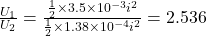## Two inductors are placed in a circuit in series with each other. Inductor 1 has an inductance of 3.5e-3 H and inductor 2 has an inductance o

Question

Two inductors are placed in a circuit in series with each other. Inductor 1 has an inductance of 3.5e-3 H and inductor 2 has an inductance of 1.38e-4 H. Anytime current is running through the inductors, what is the ratio of energy stored in inductor 1 compared to inductor 2? U1 is the energy in inductor 1 and U2 is the energy in inductor 2.

in progress 0
3 months 2021-08-02T14:52:06+00:00 1 Answers 4 views 0

Ratio of energy stored in the inductor will be 2.536

Explanation:

We have given two inductance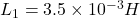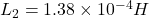As inductors  are connected in series so current will be same in both inductor

Energy stored in inductor is equal to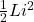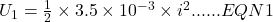Energy stored in second inductor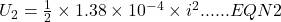Dividing equation 1 by equation 2